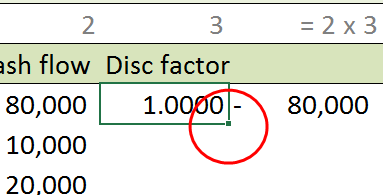# Finance example – NPV

In this module:

• Example (ABC Farming Co): net present value (NPV) in table format
• Excel pointers and the fill handle

## Net present value

An important concept in finance is the application of the Net Present Value method to project evaluation.

Example: ABC Farming Co is considering an investment of $80,000 in a new carrot washing machine, that is expected to generate the following incremental cash inflows:$10,000 (year 1), $20,000 (year 2),$15,000 (year 3), $50,000 (year 4), and$20,000 (year 5). Assume that all cash inflows occur at the end of the year. The discount rate is 5.0% pa.

Required: Calculate the net present value of the project, and present your answer in table form based on the NPV equation – $$\begin{equation} NPV=C_0 + \sum_{t=1}^{n} \frac{C_t}{(1+k)^t} \end{equation}$$ where $$C_0$$ is the initial cash flow at time zero. The future cash flows at time $$t$$ are denoted $$C_t$$ over $$n$$ periods. $$k$$ is the periodic discount rate.

Using equation 1, the solution is:

$$NPV=-80,000 + \frac{10,000}{(1+0.05)^{1}} + \frac{20,000}{(1+0.05)^{2}}+ \frac{15,000}{(1+0.05)^{3}}+ \frac{50,000}{(1+0.05)^{4}}+ \frac{20,000}{(1+0.05)^{5}} = \17,428$$

## ABC Farming Co

The task is to replicate the NPV calculation in table format as illustrated in figure 1.

The following points are a summary of the session demonstration. All cell references relate to figure 1.

### Data entry

• Leading equals sign in equation Row 36 applies index numbers to the Period, Cash flow, and Discount factor columns. Cell W36 contains a text string indicating that the column W value is the product of index 2 and index 3
• The entry in cell W36 commences with an equals (=) sign. Excel will interpret this as an equation and return an error or an information message and then attempt to return the value 6. With an equation, the multiplication symbol is a * not an x, and white space is not allowed. To solve this problem, either change the cell format to Text BEFORE the text is entered, or leave the format as General and include a leading apostrophe in the string '= 2 x 3. This is the usual method to control the operation of (=). The apostrophe appears in the formula bar, but not in the cell.
• Manually enter the individual cash flows in column index 2
• Period – fill down In the range T38:T39 enter 0 and 1. Select these two cells, then use the Fill Handle (click-and-drag down) to complete the sequence. The fill handle is discussed later in this module
• Discount factor Select cell V38 and enter the formula =(1+0.05)^-T38. The idea is to use the corresponding Period value, 0 in this case, as the exponent in the formula. $$(1+0.05)^{0}=1$$
• Period discounted cash flow – complete the formula in cell W38: =U38*V38
• Fill down index 3 and 4 – select V38:W38, then double click the fill handle to complete the two column sequence
• Sum the cash flows and discounted cash flows – use Home > Editing > AutoSum as this will automatically select the range for the SUM function
• The concatenated text and value cell In cell T46 enter the formula ="Net cash flows ="&TEXT(U44,"\$#,#"). Without the TEXT function the formula in cell T47 would return Net present value at 5% = 17427.6101399059

### Format of table

• Color format – use Home > Font > Fill Color
• Border format – use Home > Font > Borders

The completed table is shown in the Excel Web App #1 in figure 2.

## Excel pointers and the fill handle

Excel uses a number of specialised cursors or pointers for the mouse or other pointing device. In normal operation, the pointer is a open cross as shown in figure 3. Left click a cell, or click and drag when making a multi-cell selection.

To move the selection a short distance on the current worksheet, place the pointer on the border of the cell or selection. The pointer will change to open cross will change to a fine cross with arrow tips and an arrow pointer – see figure 4. Left click on the border of the selection and drag to new location.

The fill handle is use to copy or extend the selection to adjacent cells. It is the small square in the bottom right hand corner of the selection, shown in the circle in figure 5.Fig 5: Selection fill handle – the small square in the bottom right corner of the selection

When the pointer is placed over the fill handle, the pointer changes to a black closed cross – see figure 6. Either left click and drag to manually extend the selection, or double click to fill down for the length of the adjacent column vector.